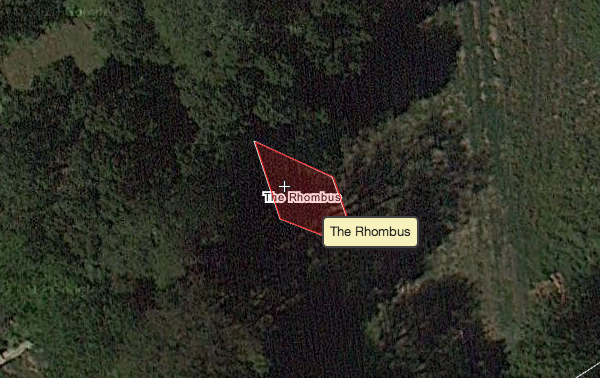# A geometry problem by Arpit Sah

Geometry Level 3The perimeter of a rhombus is 48 and the sum of the lengths of its diagonals is 26.

What is the area of the rhombus?

×